# Control Systems

Build and analyze control systems, document design decisions, and interactively evaluate controllers–all in one system, with one integrated workflow.

Underlying the Wolfram control systems solution is a powerful hybrid symbolic-numeric computation engine with numerics of any precision, high-performance symbolics, advanced visualizations, and automated algorithm selection–everything to get accurate results efficiently. The Wolfram solution is ideal for testing ideas and designing efficient and reliable control systems.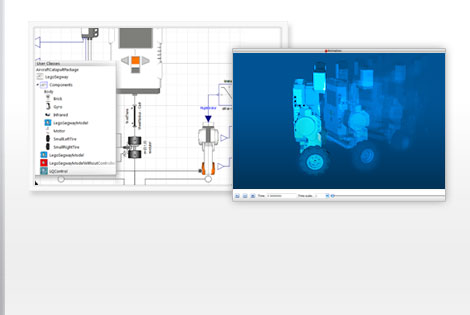Building a control system for a Segway using Wolfram SystemModeler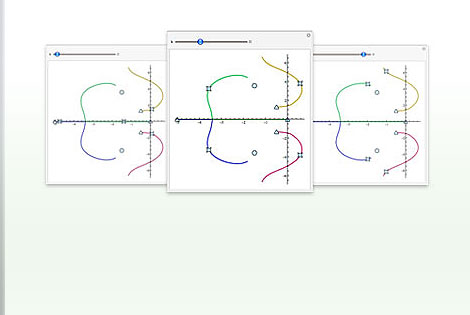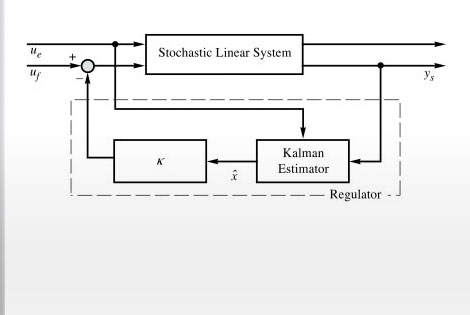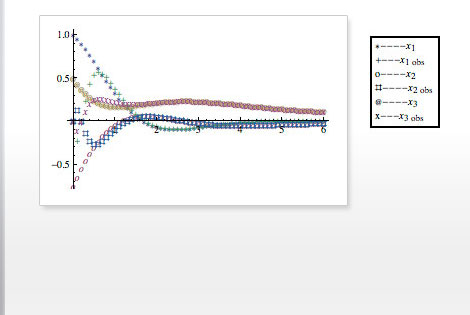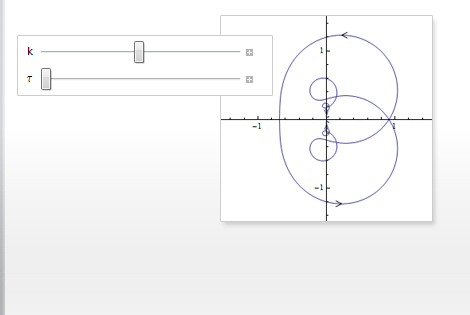The Wolfram Edge
How Wolfram Compares
Key Capabilities

Wolfram technologies include thousands of built-in functions that let you:

• Compute the state-space model of a system described by difference or differential equations and any algebraic constraints
• Analyze the stability of a system using built-in frequency-response tools, computing the poles, or solving a Lyapunov equation
• Simplify models of systems with interconnected components using block-diagram reduction
• Manipulate linear models as transfer-function or state-space data objects
• Interactively analyze the system behavior as parameters are varied
• Employ classical techniques such as Bode, Nyquist, Nichols, and root locus plots to analyze and design control systems
• Evaluate the controllability and observability properties of a system
• Compute state-space transformations to obtain decompositions that are controllable, observable, minimal, or balanced
• Obtain continuous-time equivalents of discrete-time systems for analysis and design
• Develop feedback laws to enhance the performance of dynamic systems
• Design and analyze systems with time delays and algebraic equations
• Automatically compute design quantities including closed-loop transfer functions, PID parametrizations, and more
• Estimate unmeasured states or noisy measurements
• Directly obtain models of controllers and estimators that can be easily assembled to form a closed-loop system for further simulations
• Discretize continuous-time feedback algorithms for real-time implementation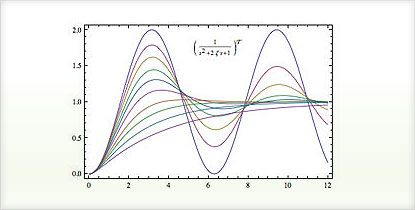Simulating the response of state-space or transfer-function models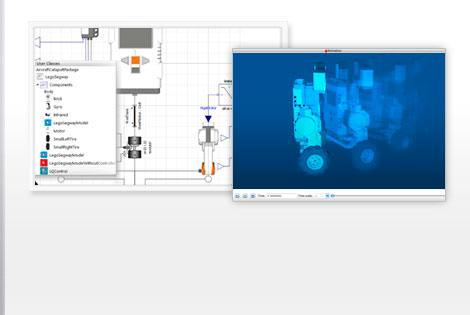Determining system stability using built-in functions

The Wolfram Edge
How Wolfram Compares
Key Capabilities

Does your current tool set have these advantages?

• Directly input both transfer-function and state-space models in natural form
Matlab allows you to specify transfer-function models only as a matrix of row vectors
• Analyze symbolic and numeric systems
Matlab handles numeric systems only
• Fully automated precision control and arbitrary precision arithmetic to ensure accurate results
Matlab and other systems relying on machine arithmetic can show critical errors due to numerical accuracy failure
• Instant interface construction to test a control system interactively for different scenarios
Unique to Wolfram technologies
• Free-form linguistic input produces immediate results without the need for syntax
Unique to Wolfram technologies
• Automated algorithm selection to get accurate results quickly—sometimes switching mid-calculation for further optimization
Other computation systems like Matlab make you analyze your equations manually to determine which function to apply
• Control systems functionality is well integrated with the core Wolfram Language and more than 20 built-in application areas, such as signal processing, time series, image processing, wavelets, linear algebra, and moreBuilding a transfer function out of a collection of poles and zeros in the complex planeSimulating a feedback control system with controller and second-order plant

The Wolfram Edge
How Wolfram Compares
Key Capabilities

The Wolfram Language includes thousands of built-in functions for computation, modeling, visualization, development, and deployment »

Control systems specific capabilities:

• Specify state-space and transfer-function models in natural form, and easily convert from one form to another
• Obtain linearized state-space models of systems described by differential or difference equations and any algebraic constraints
• Freely convert between continuous-time and discrete-time models using a wide selection of algorithms
• Perform system manipulations, such as selecting or deleting subparts, cascading a set of systems, constructing interconnections of subsystems, and more
• Analyze and design systems using frequency-response tools centered around Bode plot, Nyquist plot, Nichols plot, and singular-value plot
• Analyze state-space models and convert between different realizations, including Kalman, Jordan, balanced, and other forms

Wolfram SystemModeler is the most complete physical modeling and simulation tool for high-fidelity modeling. With SystemModeler, you can: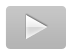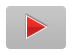— Bruce Colletti Defense Contractor Consultant

# Organizations Using Wolfram Technologies

Get your free, fully functional, 15-day trial version of Mathematica and SystemModeler via download.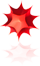### Get Mathematica

System requirements »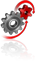### Get SystemModeler

System requirements »